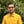Trusted answers to developer questions
Trusted Answers to Developer Questions

Related Tags

ruby
math
communitycreator

# What is Math.acos() in Ruby?Harris Amjad

Grokking Modern System Design Interview for Engineers & Managers

Ace your System Design Interview and take your career to the next level. Learn to handle the design of applications like Netflix, Quora, Facebook, Uber, and many more in a 45-min interview. Learn the RESHADED framework for architecting web-scale applications by determining requirements, constraints, and assumptions before diving into a step-by-step design process.

The acos() function calculates the inverse cosine of a number. To be more specific, it returns the inverse cosine of a number in radians. It is also called the arc cosine function.

Figure 1 shows the mathematical representation of the acos() function and Figure 2 shows the visual representation of the acos() function.

Figure 1: Mathematical representation of inverse cosine function
Figure 2: Visual representation of inverse cosine function

This function is present in the Math module.

To convert radians to degrees, use the following formula.

degrees = radians * ( 180.0 / pi )


## Syntax

acos(num)


## Parameter

This function requires a number as a parameter. The parameter must be a double value between -1 and 1, i.e., -1 <= parameter <= 1.

## Return value

This function returns the inverse cosine of a number (in radians) that is set as a parameter. The return value lies in the interval of [0, pi] radians.

## Example

#positive number in radiansprint "The value of acos(0.5) : ",  Math.acos(0.5), " Radians \n"#negative number in radiansprint "The value of acos(-0.5) : ",  Math.acos(-0.5), " Radians \n"#applying acos() and then converting the result in radians to degrees#radians = 0.5#PI = 3.14159265result = Math.acos(0.5) * (180.0 / Math::PI)print "The value of acos(0.5) : ",  result, " Degrees"

RELATED TAGS

ruby
math
communitycreator

CONTRIBUTORHarris Amjad

Grokking Modern System Design Interview for Engineers & Managers

Ace your System Design Interview and take your career to the next level. Learn to handle the design of applications like Netflix, Quora, Facebook, Uber, and many more in a 45-min interview. Learn the RESHADED framework for architecting web-scale applications by determining requirements, constraints, and assumptions before diving into a step-by-step design process.

Keep Exploring

Learn in-demand tech skills in half the time